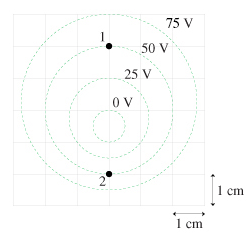# Problem: Determine the magnitude and direction of the electric field at point 2 in the figure. A. E2=(2500V/m,up) B. E2=(3750V/m,down) C. E2=(7500V/m,down) D. E2=(5000V/m,up)

###### FREE Expert Solution

Electric field:

$\overline{){\mathbf{E}}{\mathbf{=}}{\mathbf{-}}\frac{\mathbf{∆}\mathbf{V}}{\mathbf{d}}}$

ΔV = 50 - 0 = 50 V

d = 1cm (1m/100cm) = 0.01 m

82% (464 ratings)###### Problem DetailsDetermine the magnitude and direction of the electric field at point 2 in the figure.

A. E2=(2500V/m,up)

B. E2=(3750V/m,down)

C. E2=(7500V/m,down)

D. E2=(5000V/m,up)